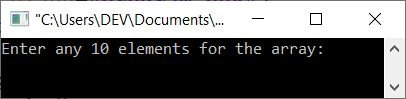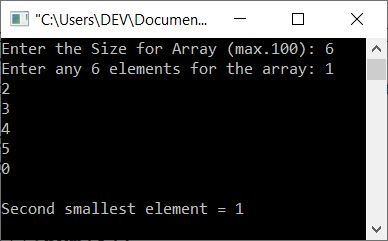# C++ Program to Find the Second Smallest Element in an Array

This article provides a program in C++ that finds and prints the second-smallest element from a given array. The first program is based on 10 array elements, where the second program is based on n array elements.

## Find the second smallest element using a for loop

The question is: write a C++ program that receives 10 array elements from the user and finds and prints the second-smallest element. Here is its answer:

```#include<iostream>

using namespace std;
int main()
{
int i, num, small, ssmall;
cout<<"Enter any 10 elements for the array: ";
for(i=0; i<10; i++)
cin>>num[i];
small = num;
for(i=0; i<10; i++)
{
if(small>num[i])
small = num[i];
}
ssmall = num;
for(i=0; i<10; i++)
{
if(ssmall>num[i])
{
if(num[i]!=small)
ssmall = num[i];
}
}
cout<<"\nSecond smallest element = "<<ssmall;
cout<<endl;
return 0;
}```

The snapshot given below shows the initial output produced by the above C++ program on finding the second smallest element from an array:Now enter the input, for example, 10, 9, 1, 2, 8, 7, 3, 4, 5, 6, as 10 array elements, and press the ENTER key to find and print the second smallest of all the given 10 array elements, as shown in the snapshot below:#### Allow the user to define the size of the array

Since the previous program was created with the limitation of only using 10 array elements, I've modified and created another program, as given below, that finds and prints the second-smallest element from an array of n  elements. The value of n and all n elements must be entered by the user at run-time.

```#include<iostream>

using namespace std;
int main()
{
int tot, i, num, s, ss;
cout<<"Enter the Size for Array (max.100): ";
cin>>tot;
cout<<"Enter any "<<tot<<" elements for the array: ";
for(i=0; i<tot; i++)
cin>>num[i];
s = num;
for(i=0; i<tot; i++)
{
if(s>num[i])
s = num[i];
}
ss = num;
for(i=0; i<tot; i++)
{
if(ss>num[i])
{
if(num[i]!=s)
ss = num[i];
}
}
cout<<"\nSecond smallest element = "<<ss;
cout<<endl;
return 0;
}```

Here is its sample run with user input of 6 as size and 1, 2, 3, 4, 5, 0 as six elements:C++ Quiz

« Previous Program Next Program »